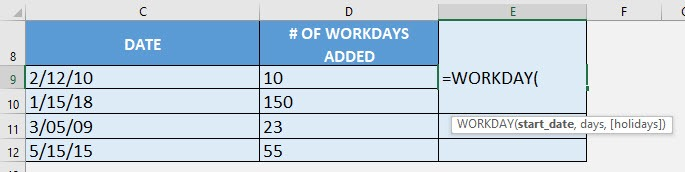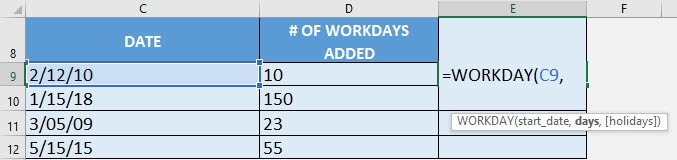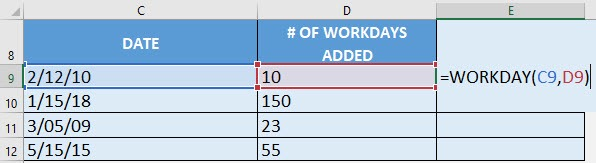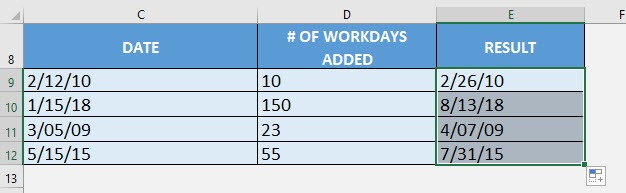What does it do?

Adds/Subtracts a specified number of workdays to a Date, which will give you a Future/Past Date

Formula breakdown:

=WORKDAY(start_date, days, [holidays])

What it means:

=WORKDAY(specified date, number of work days to add/subtract, [holidays to be considered])

If you want to calculate the future date based on a number of working days added, it will be difficult to manually calculate this!

The hard way: I would normally grab my calendar, and count the days one by one!

The easy way: Excel’s WORKDAY formula!

I explain how you can do this below:

STEP 1: We need to enter the WORKDAY function in a blank cell:

## =WORKDAY(STEP 2: The WORKDAY arguments:

## start_date

What is the specified date?

Select the cell containing the date you want to add the number of workdays to:

## =WORKDAY(C9,## days

How many work days to be added?

Select the cell containing the number of work days to be added (i.e The number of non-weekend and non-holiday days after the start date):

## =WORKDAY(C9, D9)Apply the same formula to the rest of the cells by dragging the lower right corner downwards.How to Use the Workday Formula in Excel